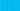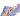PricingResources

# What Is Future Value?

Written by

Knowing how to calculate the future value of money will help you make more informed decisions when investing. Similar to time value of money, future value is a prediction that will help you estimate how the value of an investment may grow over time. While it can be simple to determine, there are some cases where calculating future value can become complicated. Here, we’ll explore the different ways you can calculate future value and when they apply.

## Understanding future value

Future value is a sum used to predict the future return on an investment, taking interest rates into account. You can use it to evaluate the viability of different investment opportunities and see which offers the most enticing level of growth. The future value equation works on the assumption that the amount you invest will go untouched on your end, but will grow through interest.

You can also use future value to predict the cost of an asset or product at a future date, if the cost increases at a constant rate. Additionally, you can use the future rate formula in reverse, to deduce what interest rate you should strive for to reach your desired growth.

You might be contemplating various savings accounts to invest in, and you can use future value of money to weigh up your options and determine which account will offer the most growth.

The calculation used to determine future value will vary depending on whether your investment offers simple annual interest or compounded annual interest.

The general future value formula assumes that the investment will grow at a constant rate through simple annual interest. To work it out, use the following calculation:

In this formula, I refers to investment amount, R refers to interest rate, and T refers to number of years.

For example, if you invested \$10,000 into a savings account that has a simple interest rate of 5% per annum, and you wanted to work out the future value of your investment in 5 years’ time, you’d do the following:

FV = 10,000 x (1 + (0.5 x 5)) = 35,000

While simple interest always applies the interest rate to the amount of the initial investment, with compound interest, the interest rate applies to the cumulative account balance for each period. So, in the above example, 5% interest would be applied to the original investment of 10,000 in the first year, but in the second, it will be applied to the total account balance, which would be 15,000 at the end of year 2.

Use this future value equation for compounded annual interest:

With our example of a £10,000 investment with a 5% compounded interest rate over 5 years, you’d do the following:

FV = 10,000 x (1 + 0.55) = 75,937.5

## Calculate future value using a financial calculator

You can save a bit of time calculating future value if you have a financial calculator with an exponential function key. Financial calculators have specialized keys that correspond with each variable in the future value formula.

Press N followed by number of years, I/YR followed by interest rate (as a whole number), and press PV followed by the total account balance being calculated, presented as a negative. Using our above example, you’d press N and 5, I/YR and 5, and PV and -10,000. Then, press PMT to indicate that there will be no further payments after the first investment, and then press FV to see future value.

## Future value limitations

Future value will only really be accurate if the interest rate stays the same and the initial investment is unchanged. Changes in the economic environment, like fluctuations in the value of an asset or currency, can impact the accuracy of the results. While it will certainly help you make more insightful investments, you should always view future value more as an approximation than a concrete figure.

#### We can help

GoCardless helps you automate payment collection, cutting down on the amount of admin your team needs to deal with when chasing invoices. Find out how GoCardless can help you with ad hoc payments or recurring payments.## Interested in automating the way you get paid? GoCardless can help

Contact sales

Sales

Contact Sales

+1(415) 523-2279

Support

help@gocardless.com

+1 (628) 241-0044